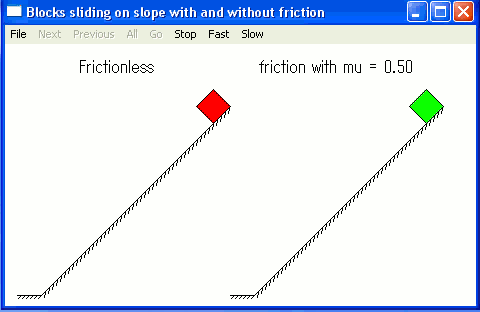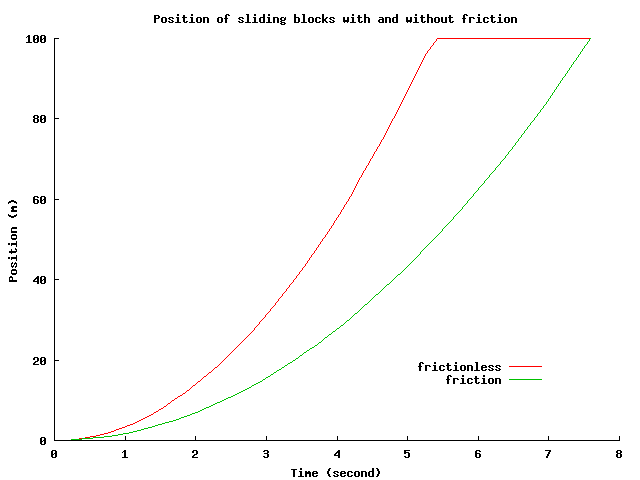Ch QuickAnimation Demos Sliding of Two Blocks With and Without Friction Problem Statement: Two blocks slide on slopes with the length of 100 meters and incline of 45 degrees. One slope has no friction and the other has the friction with the friction coefficient mu of 0.5. Plot the traveling distance of these two blocks versus time and animate their motions. Animation This program blocks_sliding.ch uses Ch QuickAnimation to animate the motion of sliding blocks shown below.Plotting The above same program blocks_sliding.ch uses Ch plotting capabilities to plot the traejctories of sliding blocks shown below